WOOCS 1.2.7.1
01270 747 008 (UK)

# An Alternative Theory Of Everything

By Raoul Selsick
toetheory@yahoo.co.uk

## Introduction

This Theory of Everything is unique in that not only in that it tries to explain a lot of physical laws with a small amount of axioms , it also includes a large range of physical ideas from many geniuses in the past. These geniuses did not always agree with one another but somehow each had a part of the truth.

Among the scientific ideas included are those of Einstein , Newton , Ampere , Gauss, Weber , Maxwell , Feynman and Purcell. The result of taking the best from each of them gives a theory which is very clear and straight forward. The basic laws of physics are strictly kept unless there is absolutely no other way of explaining the experimental evidence.

Thus the laws of ;
Conservation of energy
Conservation of momentum
Of action reaction,
are applied in a simple and direct way.

## The Underlying Principal

Magnetism and gravitation do not exist. The only forces that exist are electric forces . (I am not dealing with nuclear forces over here). The basis of magnetism and gravitation is that electric charges increase with relative velocity.

The increase in charge is q = q0 / (1 + v2 / c2)1/2 This equation is the same as the relativistic increase of mass with velocity. In fact it is the increase of charge with velocity that indirectly accounts for the for the mass increase.

This is approximately equal to q = q0 + q* 0.5 * v2 / c2

This velocity must not be measured with respect to us or the wire . We must measure the velocity of one charge w.r.t. another. It is this increase which accounts for what we call magnetism and gravitation. The velocity always has to be calculated w.r.t the moving charges themselves and not with respect to us or the wires. In any small amount of matter the charges are enormous. Any slight unbalance results in large resultant forces.

# Magnetism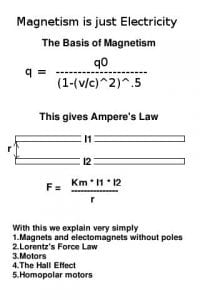Magnetism does not exist. A current consists of stationary positive charges and moving negative charges. Let us start with two parallel wires with an equal current in the same direction. We must consider four basic charge-forces. Each wire has a fixed positive charge and a moving negative charge. We must consider the sum of the four forces between the two wires. We have the positive in the first to the positive in the second , the positive in the first to the negative in the second. We also have the negative in the first to the positive in the second and the negative in the first to the negative in the second. The positive-positive have zero relative velocity and so do not change. The negative-negative charges move at the same velocities and so the force between them is the same. The positive in one wire to the negative in the other wire have a relative velocity and are therefore a tiny bit stronger. Likewise the negative in the first to the positive in the second have a relative velocity as above.

Normally the positive-negative and negative-positive exactly balance the positive-positive and negative – negative. The positive-negative in a moving current are now stronger and thus the two wires will attract one another. The total charges in the wire are so big that even a slight imbalance causes a big force, i.e. the magnetic force between the wires.

If the currents now goes in opposite directions the negative-negative charges will now move at at 2 v. The force between the two negative charges now increases by v2 and is so 4 times as strong. The positive-negative and negative-positive are thus less and the wires will repel one another.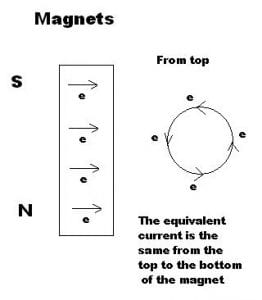Magnets are only perpetual electric currents. If we carefully note the direction of the currents we will notice something like the two poles.

There will still be a big difference. If we place a small cylindrical magnet next to a longer one. We will place the magnets n-s, s-n so that they attract one another . They will be pulled together. Stuck directly one to another. If there was two poles
the smaller magnet’s pole should be pulled upwards towards the larger’s pole. There is no vertical force on the two magnets. We do not have forces between two poles . The forces that exist are those between two sets of virtual currents going around the circumference of the magnets.

The magnets “poles” are more accurately described in terms of the virtual currents than in terms of two “poles”.

This is a proof that the virtual currents going in the same directions attract one another and that there are no poles. Poles are simply an illusion and do not exist. Looking for any magnetic poles is absurd as even dipoles do not exist. It is just as stupid as looking for the edges of the earth. Magnetic poles are simply an historical anomaly.

To see this clearly one just needs to carefully apply Ampere’s two wire current law. Like currents attract unlike repel.

Various Magnetism examples I will not go into any long explications over here. In most cases all we need is the result which is Ampere’s law : currents going In the same direction attract and in the opposite repel .I have used square coils instead of normal circular ones so that the forces involved will come out clearer.

Please note that I will also use the electron flow instead of the current flow. Thus either use the left hand law to find
the “historical north pole” or change the direction around to get the current before you apply the right hand law.

## The Attraction and Repulsion of two Magnets

Lorentz’s Law: F = E * q + B * v * q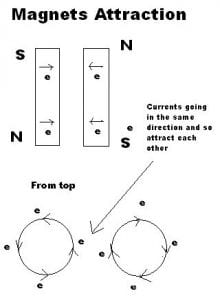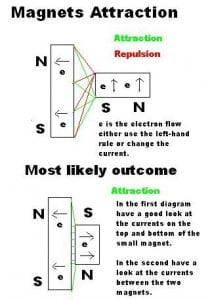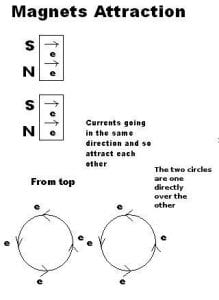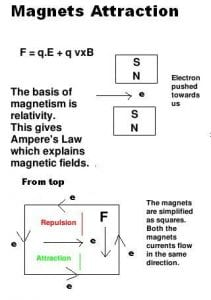##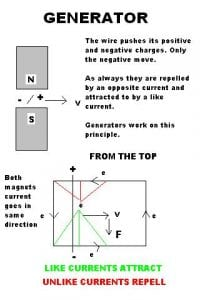Generators

Motors work in a similar way. Just imagine a current moving through the wire.

Apology
I am sorry for using a flow which “goes around the wrong way” ,but if historically the flow was correct there would not be so much confusion about all of this and we would have seen all of this a long time ago!

# Gravitation

As everyone knows matter consists of atoms having a fixed positive nucleus with electrons moving around it. We will consider the various forces of two fixed bodies in space.

Positive-positive have no relative velocity thus no increase in charge. Positive-negative and negative-positive a relative
velocity and thus an increase in charge resulting in an attractive force. For negative-negative the force-balance will sometimes be positive sometimes negative and sometimes zero. It all depends on where in the circle the two electrons
are. On average the total will be less than all the positive-negative and negative-positive forces.

The result is a very slight attraction.

Thus magnetism and gravity do not really exist and are only true relativistic corrections to the simple electrostatic forces.

## Acceleration of charge

Up till now I have dealt only with moving charges that do not accelerate.The acceleration of charges opens up another extremely interesting area. Any accelerating charge must be balanced by an equal and opposite acceleration.

This can be applied in one of two ways. Either the accelerating charge will accelerate a like charge in the opposite direction or an opposite charge in the same direction. This is the general rule of which many fundamental rules of physics are directly derived.

These laws include ;
Newton’s law of to every action there is an equal and opposite reaction. Newton’s law is a direct application of this simple law applied to composite charges.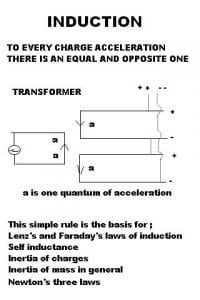Lenz’s law of induction
Changing currents are accelerating charges. These charges will cause an acceleration of the charges (i.e. Electrons ) in a nearby wire in the opposite direction. Transformers are directly based on this rule. Electronic coils are just the accelerating charges acting directly on themselves.

The Mach principal of inertia
Matter is composed of charges the acceleration of which causes a mutual acceleration with all other charges in the whole universe. Inertial mass is simply a complex integrated example of electronic coils.

## Light and All other Electromagnetic Radiation

Light does not exist . No one has ever seen light and no one will ever see light . What we call light is simply a quantum
Balance of energy-momentum. Seeing is the result of various electric currents arriving from our eye to our brain .

Just like a meticulous bookkeeper must account for each and every cent so the universe must keep an account in each and every energy-momentum reaction in the universe.

All energy and momentum must be entirely accounted for, for every action-reaction in the whole universe. If a certain reaction we are studying has a certain imbalance in its energy-momentum this must be balanced by another reaction
at time d/c somewhere else in the universe. D is the distance of the second reaction to the first.

The exact position that this will occur can only be determined statistically by means of least path analysis etc much like that of Feynman. This process is dynamic and takes into account any changes in the universe between the imbalance of the first reaction until the balance of the second.

Over here we have Feynman’s explication for a light path. In my theory this is not a photon’s path but it is more like a dynamic field. If some energy-momentum is lacking at some point in space it must be somehow corrected . A dynamic field is set up which must be somehow satisfied. The point at which it will be corrected corresponds exactly to Feynman’s calculation.

After it has been corrected we claim a “photon was emitted from A and received by B”.

Some Simple Rules
Higher energy quantum goes in straighter lines and quantum (i.e. the photons) will be blocked by obstacles greater than there wavelength ie. lambda. (This is basic physics restated in a different way.)

What we call seeing can thus be described in the following way ;

I will describe a case where we observe directly a light source , but it can be easily extended to the more general cases .

A lit candle contains heated particles accelerating rapidly in all directions. In many of the collisions we will under certain circumstances have an imbalance in the energy-momentum. Some of this energy-momentum imbalance will be corrected in the eye of the observer.

The electrons emitted will finally travel to the brain …

Electrons accelerating in a transformer coil will “emit photons”.These “photons” will “accelerate” other electrons in the other coil. Electrons hitting a led plate “emit X-rays”. These X-rays will hit a metal plate and cause the Einstein
electron emission.

Two applications of this energy-momentum conservation.

## The Doppler Effect

The Doppler effect is a currently observed shift of electromagnetic radiation frequency due to relative movement. According to our current understanding of physics the Doppler shift does not make much sense. When light was considered moving in an ether it was logical, but, without an ether it becomes fairly complicated.

By applying the conservation of momentum we could obtain the “Doppler Equations” in a simple and straight forward way .These equations are not exactly the same as those in our current text books but I claim that my results are not any less accurate than those in our current text books. The results are very much the same and I claim that all observations
fit my equations just as well.

## The Redshift Calculation using the Momentum Conservation

If we consider light as a dynamic field that just corrects any unbalance of energy-momentum the redshift is straight away clear and obvious. Assume a moving body Alice fires a “photon” at a fixed body called Bob. The momentum before must equal the momentum afterwards;

Thus the momentum received must be equal the momentum emitted plus the momentum due to the velocity of Alice. We also have to take into account the momentum of the mass itself that was converted into the “electromagnetic wave”.

h * f/ c = h * fe / c + (h * fe / c / c) * -v

The second term is the momentum of the mass that was converted into light.

This reduces to;
fo= f* (1 – v / c)

and finally;
Lo= L/ (1 – v / c)

This is fairly close to the relativistic redshift calculation when v/c is fairly small (i.e. <1/10).

## The Gravitational Red-Shift

Einstein predicted and carefully explained using his general theory of relativity that light going from one gravitational potential to another should change its frequency . This was observed by Pound and Rebka.

Using a strict conservation of energy we can obtain the gravitational red-shift. If we only use the following facts we can derive the gravitational redshift;

1. The conservation of energy-matter
2. The potential energy on earth PE = m * g * h (or that of a star G * M / r )
3. The energy of a photon E = h * f
4. E=mc2.

There are no other theories or facts that we need to prove the gravitational redshift. To show this we make a thought experiment with Alice and Bob.

Alice and Bill have decided to do something dishonest. They want to break the first law of physics that prohibits
perpetual motion machines. Alice goes to the bottom of a mountain and Bill to the top.

Alice will then convert 1kg of water to a laser light and flash it up to Bill. Bill will then convert this laser light back to water and let it flow down the mountain. They will then sell the energy of the flowing water on the black market .

This is obviously wrong.

The reason is that the potential energy of the water must be subtracted from the energy Bill can get from the light rays. The energy before must be equals the energy afterwards.

Thus;

The energy received must equal the energy emitted minus the energy due to the potential energy of the water.

h * f= h * fe – (h * fe / c / c) * g * h

This reduces to;
f= f* (1 – g * h / c / c)

This is Einstein’s result at low gravitational forces.

It is accepted by scientists that at normal gravitational potential differences this can be explained by a conservation of energy. They claim that at low gravities a Newton approach approximates the red-shift.

No black hole or complicated mathematics will change this basic fact of physics.

I claim that Einstein can bend the whole universe around but only a pure experimental proof without any other possible explications repeated many times could change my claim that energy-momentum is always meticulously conserved.

Just like any scientist would violently deny any attempt to make a perpetual movement machine by pumping water
with the electricity generated when it flowed down a hill so I claim energy must always be meticulously kept. If
a big company claimed that because they are so big they do not need to account for the millions they siphoned off into their private accounts they would straight away be arrested so over here;

I claim that energy must always be meticulously kept.

I claim my equation having a strict conservation of energy is right and that of Einstein is approximate.

If light ( and all other electromagnetic radiation ) could be considered as an energy-momentum conservation it would open up many interesting avenues in our understanding of optics.

## Future Applications

Millions of good dollars are spent on research . I believe that a little should be given to understanding the exact nature of magnetism.

Currently the Maxwell-Faraday approach dominates the scientific community. A few authors (see the Link section) have have attempted to approach magnetism differently.

It seems it is extremely difficult to accurately test the various approaches to magnetism. There are many parasite effects that are really difficult to get rid of. Nevertheless a careful methodical approach should enable us to determine if not the right set of formulae at least those which are the most accurate.

It could be possible that another set of formulae are more accurate which Would open up new possibilities for research and development.

## Related Links

http://www.ifi.unicamp.br/~assis/Pramana-J-Phys-V55-p393-404(2000).pdf
Assis quotes Weber here who over here has another set of equations. Comparison between Weber’s electrodynamics and classical electrodynamics

http://www.df.lth.se/~snorkelf/Longitudinal/node4.html
Lars Johansson studies various force equations. Maxwell’s equations do not obey Newton’s law of to every action there is an equal and opposite reaction for each current element. When considering the whole circuit they do. The other formulae obey Newton’s law even for every current element.

http://www.andrijar.com/rwoteewdm/index.htm
A large collection of electromagnetic formulae.

http://physics.weber.edu/schroeder/mrr/MRRtalk.html
Schroeder explains the approach of a Nobel prize winner Purcell who also claims magnetism is just a relativistic effect. His
approach is different to mine.

http://www.21stcenturysciencetech.com/articles/spring01/Electrodynamics.html
Various discussions about Weber and Gauss. They already proposed the idea that gravitation was due to electrostatic forces. Weber also considered magnetism as the result of sum of the four forces in the two wires.

If the results of the experiments presented here are accurate and still apply to our current knowledge where only electrons move I would have to add on a direction term to my equations.

To quote the article;
“However, Weber’s unifying approach was to assume that the relative velocities of the electrical particles produced a modification in the Coulomb electrostatic force, to produce the resultant force between the wires.”
(…)
“Here it is the force, rather than the mass, which varies with the
relative motion. “

My theory claims that it is the charge that varies.

The article continues;
“Mossotti, a French physics teacher at the University of Buenos Aires. Mossotti proposed to account for gravitation in the following way: If matter is assumed to be constituted of equal amounts of positive and negative electricity, then, by the usual interpretation, there would be a cancellation of the attractive and repulsive forces. However, if it be assumed that the attractive forces between particles of opposite electrical charge, slightly exceed the repulsive forces of the like particles, a universal tendency for attraction would result.”

“Weber gave serious consideration to the Mossotti hypothesis. In a posthumously published manuscript on the relationship of electricity and gravitation, he discussed the extreme difficulty of experimentally determining whether such a small difference between attractive and repulsive forces exists.”

Ampere Tension and Newton’s Laws
http://redshift.vif.com/JournalFiles/Pre2001/V00NO17PDF/NR17PHI.PDF
Ampere claimed that two adjacent current elements in a circuit should repel each other. This article shows how difficult it is to test this hypothesis. According to my theory under a constant current they should attract but under certain conditions with a changing current they would repel.

http://www.mathpages.com/home/kmath527/kmath527.htm
Over here the author claims Weber had solved the problem Einstein became famous for the perihelion of Mercury. He explained it using a logic similar to my theory.
“At about this same time, theoretical physicists such as Gauss and Weber were investigating modifications of the Coulomb inverse-square law by introducing a velocity-dependent potential to represent the electromagnetic field, consistent with the finite propagation speed of changes in the field (i.e., the speed of electromagnetic waves). It was found that this same type of law, when applied to gravitation, predicted perihelion advance for the two-body problem on the same order of magnitude as actually observed for Mercury.”

Next Page: Fitzpatrick’s Theory Of Everything
Previous Page: Other Theory

## 2 Comments

1.From the theory we can actually calculate the magnetic force from first principles.In other words from relativity and Coulomb’s law we get magnetism (Here magnetism only refers to the static type and not the magnetism of accelerating charges and induction).

The result is virtually the same as the classical Maxwell or Biot-Savart formulas!!!

There is still one important theoretical difference.The classical method breaks Newton’s action-reaction law for current elements.Maxwell claimed that the ether somehow “absorbed” the and transformed the force.

On the attached image I compare the current element force formula according to Maxwell and according to my calculations.

The formulas are virtually the same except for two points ;
1.The angle to be used and the direction of the resultant force.
According to my formula the force is always along the line joining the two elements.Newton’s law will thus be strictly kept.2.If I understand what you are saying magnetism is basically an application of Ampers current law. How do you explain the magnetic torque and magnetic motors ? How can they turn around ?

Comments are closed.